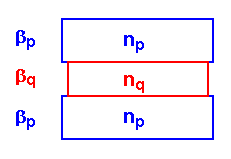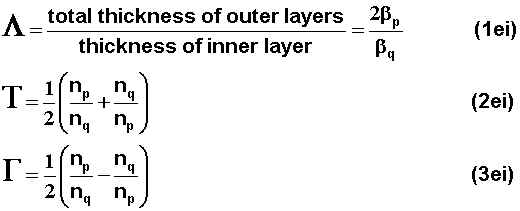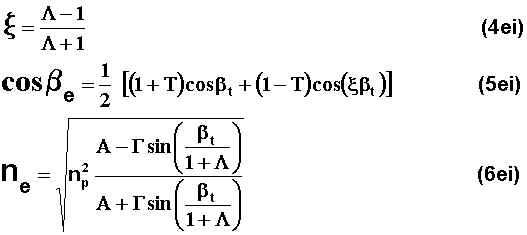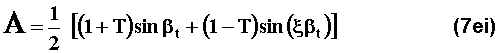Thin Film Design & ApplicationsEquivalent Layers

There are several methods to calculating the equivalent index of a symmetrical period of layers.  For this course we will focus on the most common, the three layer stack.

This calculation applies to any nonabsorbing symmetrical group of layers.   The layout of the stack would look like the following figure:where,Web www.kruschwitz.com